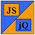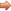# JavaScript & jQuery Tutorials

JS HomeJS ReferenceMathasin()

## asin()`Math`  class method

### Description

Returns the arcsine of a number in radians between -`PI`/2 and `PI`/2.

• Radians to Degrees - divide number by (`Math.PI` / 180).
• Degrees to Radians - multiply number by (`Math.PI` * 180).
• Like all class methods `asin()` should be used on the class rather than an instance of the class.

### Syntax

Signature Description
`Math.asin(value)`Returns the arcsine of a number in radians between -`PI`/2 and `PI`/2.

### Parameters

Parameter Description
`value`The value you wish to find the arcsine in radians for:
• A number in the range -1 to 1.
• Values outside this range return `NaN`.

### Examples

The code below displays` asin()` usage.

``````
// Store results in an array.
var asinValues = new Array(5);
asinValues = Math.asin(-1);
asinValues = Math.asin(0);
asinValues = Math.asin(0.75);
asinValues = Math.asin(-2);
asinValues = Math.asin('a string');
asinValues = Math.asin();